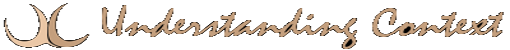# 27 Mar standard deviation

Standard deviation is a formula for measuring the distance from the center of the average result. It is the most commonly used indicator of degree of dispersion and is the most reliable measure for estimating the variability in a population. Standard deviation can be extremely useful in the context of discriminating between normal, exceptional and anomalous cases. The formula for standard deviation is the square root of the mean of the squared deviations from the mean of the distribution.
Keywords: exception
range
statistical analysis
probability
References: algorithms
automata
computing
logic
rules

This site uses Akismet to reduce spam. Learn how your comment data is processed.